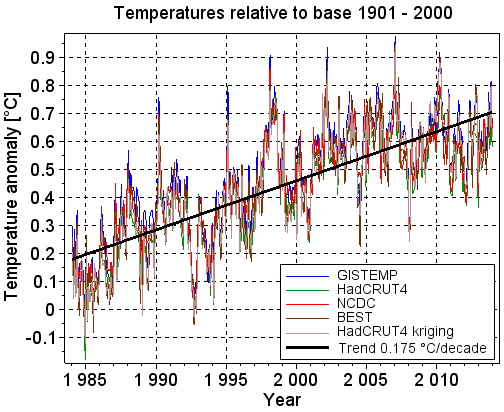## mandag 16. juni 2014

### Linear regression analysis

This is the first blog post in a series of five that analyse trends in the global surface temperatures. The posts put emphasis on the mathematics and the statistics used in the analyses. The posts are numbered 1 to 5. They should be read consecutively.

Post 1    Linear regression analysis
Post 2    Hypothesis testing of temperature trends
Post 3    Confidence intervals of temperature trends
Post 4    Statistical power of temperature trends
Post 5    Piecewise linear regression applied to temperature trends

The posts are gathered in this pdf document.

Start of post 1 Linear regression analysis

The colored lines in Figure 1.1 show the monthly temperature anomalies from January 1984 to December 2013 for five different temperature series. A temperature anomaly is the difference between the real temperature and the average temperature. A base period is a time interval in which the average temperature is calculated. For brevity we often write temperature instead of temperature anomaly. The five series contain the global land and ocean surface temperature anomalies.Figure 1.1: Temperatures in the last 30 years for five different temperature series. The trend line is calculated based on the average of the temperature series.

The HadCRUT4 temperatures are anomalies relative to the base period 1961-1990. As an example, the HadCRUT4 temperature in January 2014 is 0.507°C, telling that the temperature in that month was 0.507°C warmer than the average of the January temperatures in the base period. The temperature series apply different algorithms to calculate the anomalies, and some of them use other base periods than HadCRUT4 does.

The base periods of the temperature series in Figure 1.1 are modified so that they all are from January 1901 to December 2000. This is done to make the temperatures compatible. The figure shows that there is little difference between the five series. Analyses based on the HadCRUT4 series are therefore representative also for the other series, and we will therefore focus on the HadCRUT4 temperatures in the next posts.

The thick black line in Figure 1.1 is the trend line of the average of the five temperature series. The trend line is calculated with linear regression analysis, and it is the best fit to the average of the temperatures. It shows that the temperature has been increasing with 0.175°C per decade during the last three decades.

Mathematics

A linear trend line is a simple model of the connection between an independent variable X and a dependent variable Y. In our case X is the time and Y is the average of the five temperature series.

a is the intercept between the trend line and the Y axis, and b is the slope of the trend line.

Linear regression analysis uses a set xi yi measurements to estimate a and b so that the resulting trend line is a “best fit” to the measurements.

Each yi measurement deviates more or less from the trend line. The vertical distance ei between the yi measurement and the trend line is treated as the error (residual) of the measurement.

The hat symbol above a and b means the estimate of those values.

SSE is the Sum of the Squared Errors.

The estimates of a and b are the values that minimize SSE. Therefore the first derivatives of SSE with respect to the estimates of a and b shall both be zero.

(1.6) solves (1.5) with respect to b
The last line in (1.6) shows how to estimate the slope. The estimate of the slope must be inserted into the last line in (1.4) to calculate the estimate of the intercept.

Slope expressed as covariance divided with variance

The estimate of the slope b may be expressed as the covariance between X and Y divided with the variance of X. The rest of the post derives this connection.

The variance of X is a measure of the variations in X, as shown below. The operator E means Expected value of.

(1.7) shows that the denominator in the estimate of the slope (1.6) is the variance of X.

The covariance between the variables X and Y is a measure of how they change together. It is zero if they change independently of each other, it is positive if they tend to change in the same direction, and it is negative if they tend to change in the opposite direction.

(1.8) shows that the numerator in the estimate of the slope in (1.6) is the covariance between X and Y. The estimate of the slope may therefore be rewritten as

The first line in (1.9) gives an intuitive explanation of the slope, and it is easy to remember. The last line in (1.9) is simpler to program than the last line in (1.6).

Reference for the mathematics

Hans von Storch, Francis W. Zwiers. 2001. Statistical Analysis in Climate Research is our main reference. Chapter 8.3 'Fitting and Diagnosing Simple Regression Models' explains the mathematics of linear regression analysis.

Next post in the series